## COUNTIF Mastery: 8 Essential Excel Examples You Need Know

In daily work, counting the occurrences of specific criteria in a large Excel dataset can feel like searching for a needle in a haystack. But do not worry, Excel's COUNTIF function is here to rescue you. The COUNTIF function is a powerful tool that enables you to quickly and accurately count the number of data points that meet specific criteria. In this article, we delve into the syntax and usage of the COUNTIF function, providing eight examples to help you harness the full potential of this powerful analysis function.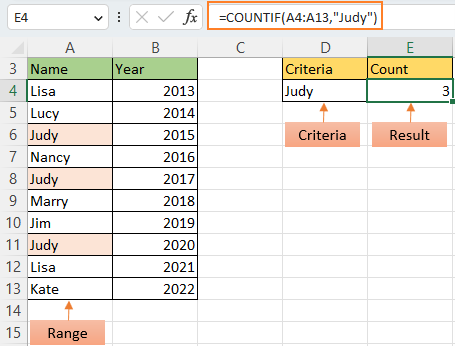### Video: COUNTIF - 8 Essential Excel Examples

Subscribe our channel now: unlock top-tier Excel tips!

### COUNTIF function: syntax and arguments

COUNTIF function is used to count the number of cells in a range that meet a condition.

Syntax

The generic syntax for COUNTIF looks like this:

``=COUNTIF(range, criteria)``

Arguments

• Range: Required. This is the range in which you want to count the specific criteria.
• Criteria: Required. This defines what you are looking for within the defined range.

A simple example for understanding the COUNTIF function

To look for a name “Judy” in a range A4:A13 and count, you should use the COUNTIF function as below:

``=COUNTIF(A4:A13,"Judy")``

Then press Enter key to get the count.Here we list seven scenarios below for you to better understand how to use COUNTIF function.

### COUNTIF basic usages

#### Count if cells with specific text (exact match)

The COUNTIF function can be used to count cells which are equal to a specific number, text or date.

Here we take the table A3:C13 as an example, column A contains fruit names, column B contains numbers, and column C contains dates. We'll separately count the occurrences of 'Apple', '100', and '6/10/2023' within these columns.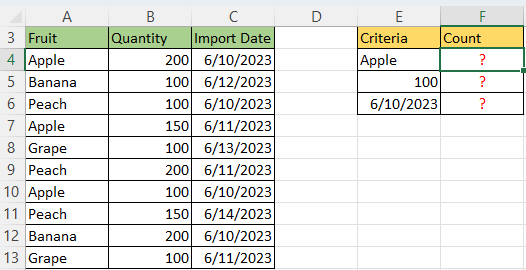The table below provides 3 different scenarios based on above example to show how to write the criteria in the COUNTIF function:

 Target Criteria Formula Cells equal to Apple "Apple" =COUNTIF(A4:A13,"Apple") Cells equal to 100 100 or "100" =COUNTIF(B4:B13,100) Cells equal to 6/10/2023 "6/10/2023" =COUNTIF(C4:C13,"6/10/2023")

After putting the COUNTIF function, press Enter key to get the counted result.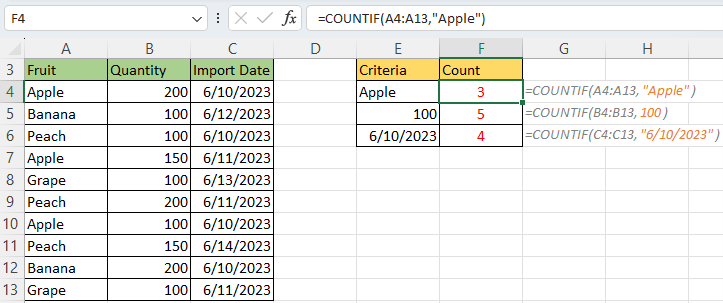Notes:
• A word or several words or a date should be enclosed with quotas as criteria.
• Instead of typing criteria, you can use a reference to any cell containing the criteria and get the same results, e.g. =COUNTIF(C4:C13,E6).

Craving an easy way to count, select and highlight cells matching specific text? Kutools for Excel has the answer with its Select Specific Cells feature! Experience its power and simplicity firsthand by downloading Kutools for Excel and start your 30-day free trial today.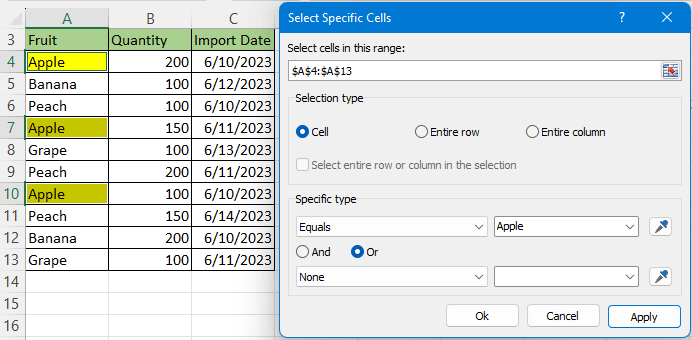#### Count if cells contain specific text (partial match)

If you want to count cells that contain specific texts, the COUNTIF function can also help you by utilizing wildcard characters.

Wildcard characters:

• A question mark (?) matches any one character, for example, L??? finds "Lisa" and "Lucy".
• An asterisk (*) matches zero or more characters of any kind, for example, *cy finds "Lucy" and "Nancy".
• The tilde (~) is the "escape" character, putting it before a wildcard (such as ~*,~?,~*), the wildcard is then treated as a regular character, for example, ~* finds "*".

In this section, we will take counting the occurrences of the text begins with "L" in the column A3:A13 as an example.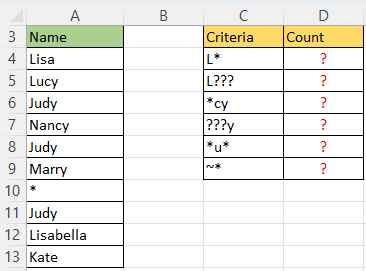And here, we present a list of common scenarios you may encounter based on the instance, along with the corresponding criteria that should be used in the COUNTIF function, as well as the formulas required.

 Target Criteria Formula Cells start with L "L*" =COUNTIF(A4:A13,"L*") Cells start with L and end with any 3 characters "L???" =COUNTIF(A4:A13,"L???") Cells end with cy "*cy" =COUNTIF(A4:A13,"*cy") Cells start with any 3 characters and end with y "???y" =COUNTIF(A4:A13,"???y") Cells contain u "*u*" =COUNTIF(A4:A13,"*u*") Cells equal to * "~*" =COUNTIF(A4:A13,"~*")

After putting the COUNTIF function, press Enter key to get the counted result.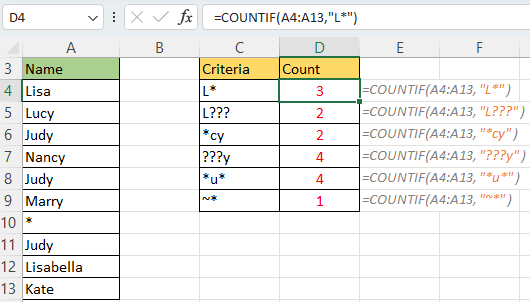Note: Instead of typing criteria, you can use a reference to any cell containing the criteria and get the same results, e.g. =COUNTIF(A4:A13,C4).

Looking to light up those Excel cells that hold specific text? Kutools for Excel is your secret weapon! The Select Specific Cells feature will do the job in no time. Get a taste of efficiency by downloading Kutools for Excel - start your 30-day free trial and watch your productivity soar!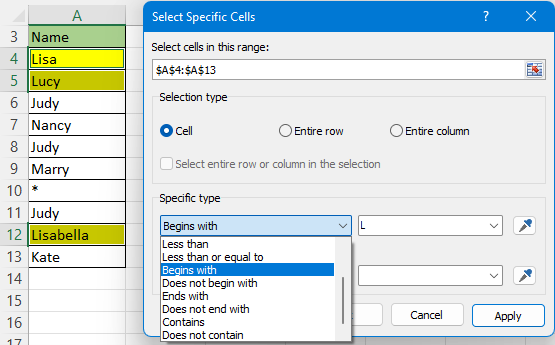#### Count if cells are not blank or blank

If you have a large range of data and need to efficiently count the number of empty or non-empty cells, the COUNTIF function will be a valuable aid.

Here, we will use the range A4:A11 as an example to count the not empty cells and empty cells.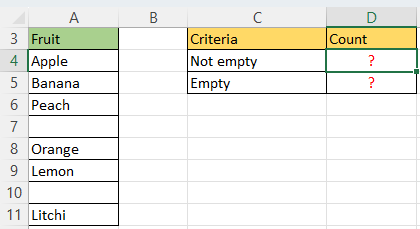Target Criteria Formula Cells are non blank "<>" =COUNTIF(A4:A11,"<>") Cells are blank "" =COUNTIF(A4:A11,"")

After putting the COUNTIF function, press Enter key to get the result.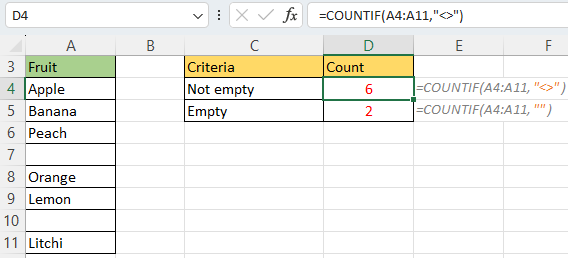Get ahead in Excel! With Kutools for Excel's Select Nonblank Cells feature, count and highlight non-empty cells in an instant. Try Kutools now with a 30-day free trial and boost your productivity!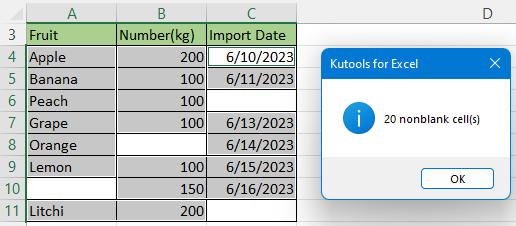#### Count if cells contain texts or numbers only

Sometimes, you may need to count cells that contain text strings or numbers only. In such instances, the COUNTIF function can come in quite handy.

Here, we will use the range A4:A11 as an example to count the cells that contain text only or number only.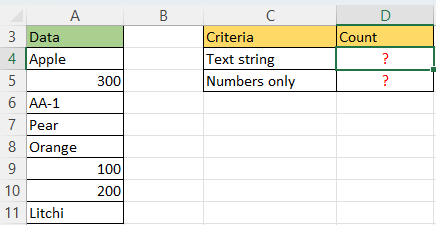Target Criteria Formula Cells contain text string "*" =COUNTIF(A4:A11,"*") Cells contain numbers only "<>"&"*" =COUNTIF(A4:A11,"<>"&"*")

After putting the COUNTIF function, press Enter key to get the result.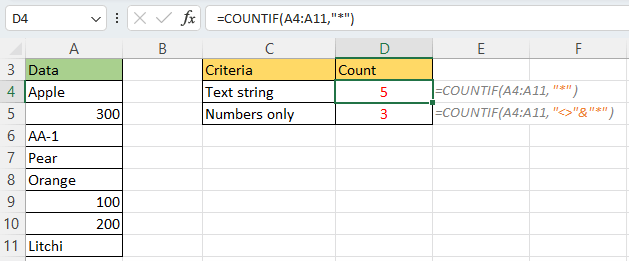#### Count if numbers are greater than/less than/equal to

To count cells with values in range A4:A13 greater than, less than, or equal to a specific number, the COUNTIF function also can help you.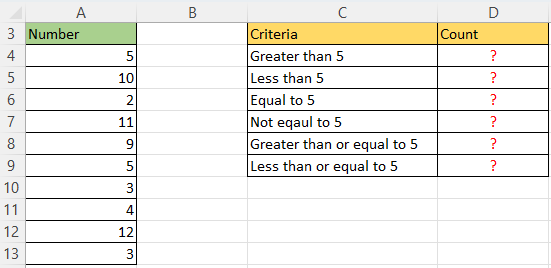You can simply add the corresponding operator to the criteria. The table below illustrates the operators you can use.

 Target Criteria Formula Values greater than 5 ">5" =COUNTIF(A4:A13,">5") Values less than 5 "<5" =COUNTIF(A4:A13,"<5") Values equal to 5 "=5" =COUNTIF(A4:A13,"=5") Values not equal to 5 "<>5" =COUNTIF(A4:A13,"<>5") Values greater than or equal to 5 ">=5" =COUNTIF(A4:A13,">=5") Values less than or equal to 5 "<=5" =COUNTIF(A4:A13,"<=5")

Press Enter key after inputting the COUNTIF formula.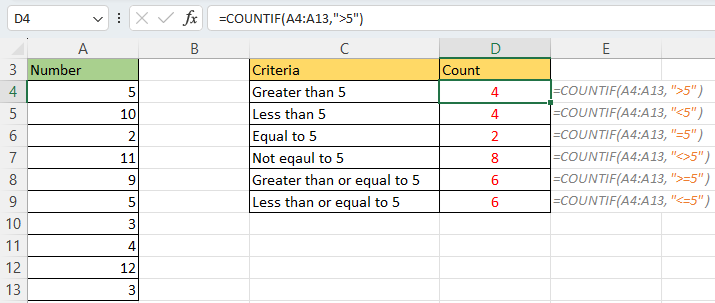Notes:
• It's important to note that in COUNTIF formulas, operators with numbers should always be enclosed in quotes.
• Instead of typing criteria, you can use a reference to any cell containing the criteria and get the same results, e.g. =COUNTIF(A4:A13,C4), C4 contains the criterion >5.

#### Count if dates are greater than/less than/equal to

If you need to count cells with dates that are greater than, less than, or equal to a specific date, you can use formulas similar to the ones we discussed earlier. The same principles apply to dates as they do to numbers. Here are a few examples to illustrate:

 Target Criteria Formula Dates greater than 6/17/2023 ">6/17/2023"" =COUNTIF(A4:A13,">6/17/2023")) Dates equal to 6/17/2023 "=6/17/2023" =COUNTIF(A4:A13,"=6/17/2023") Dates less than or equal to 6/17/2023 "<=6/17/2023" =COUNTIF(A4:A13,"<=6/17/2023")

Press Enter key to get the count after putting the COUNTIF formula.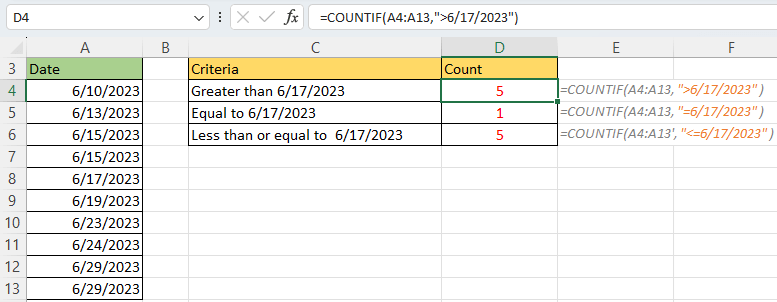In addition to the common uses we discussed, you can leverage the COUNTIF function in combination with specific Excel Date and Time functions, such as TODAY function, to count cells based on the current date. This allows you to dynamically track and count cells that meet the criteria of the current date. Here are some examples of how you can use the COUNTIF function with TODAY():

 Target Criteria Formula Dates greater than today ">"&TODAY() =COUNTIF(A4:A13,">"&TODAY()) Dates equal to today "="&TODAY()) =COUNTIF(A4:A13,"="&TODAY()) Dates less than a week from today ">"&TODAY()-7 =COUNTIF(A4:A13,">"&TODAY()-7)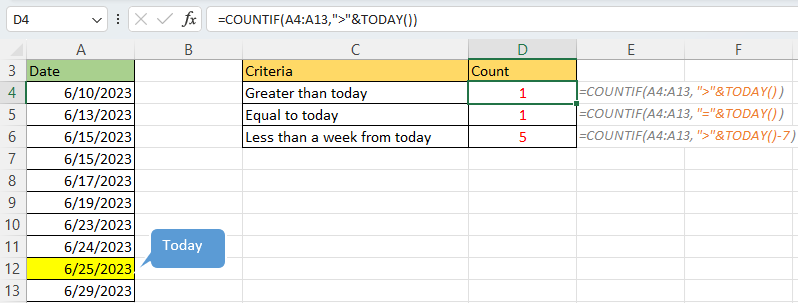#### Count if cells with multiple criteria (or/and)

Indeed, the Excel COUNTIF function is not specifically designed to count cells with multiple criteria. In such cases, the recommended function is COUNTIFS function. However, there are certain situations where you can achieve the desired result by combining multiple COUNTIF functions within a single formula.

>> Or criteria

Supposing you want to count the cells that equal to “Peach” or “Apple”, you should combine two COUNTIF functions together as below shown:

``=COUNTIF(A4:A11,"Peach")+COUNTIF(A4:A11,"Apple")``

Press Enter key to get the count.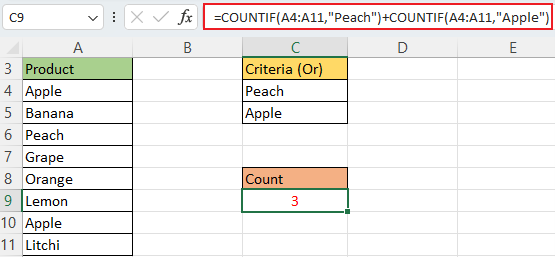Note: Instead of typing criteria, you can use a reference to any cell containing the criteria and get the same results, e.g. =COUNTIF(A4:A11,C4)+COUNTIF(A4:A11,C5).

Please note that in this scenario, we use the plus sign (+) to combine the results obtained from two COUNTIF functions. Similarly, you can create a COUNTIF formula with multiple conditions using the same approach. Here's an example of a COUNTIF formula with three OR conditions that counts occurrences of "Peach", "Apple" and "Lemon":

``=COUNTIF(A2:A9,"Peach")+COUNTIF(A2:A9,"Apple")+COUNTIF(A2:A9,"Lemon")``

>> And criteria

Supposing you want to count the numbers that are greater than 5 and less than 10 in a range A4:A11, you can combine two COUNTIF functions as below screenshot shown:

``=COUNTIF(A4:A11,">5")-COUNTIF(A4:A11,">=10")``

Press Enter key to get the count.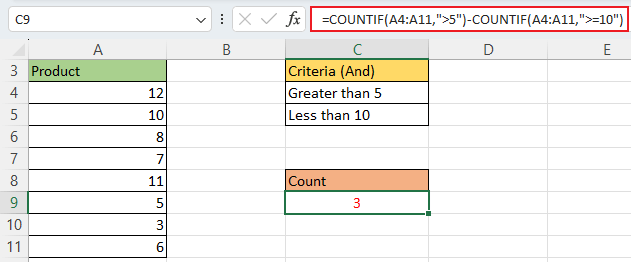Please note that in this scenario, we use the minus sign (-) to combine the results obtained from two COUNTIF functions.

#### Count if cells are unique or duplicate values

If you want to count unique values or duplicate values in the range A3:A13, the COUNTIF function also can help you.

##### Step 1: Identify whether each value is unique or duplicate
1. In the column next to the values you want to count, use the formula below to identify the related value is unique or duplicate, TRUE indicates the related value is duplicate, FALSE means the value is unique.
``=COUNTIF(\$A\$4:\$A\$13,A4)>1``
2. Press Enter key and double click on the fill handle (the small green square in the lower-right corner in the first result cell), then all results are shown.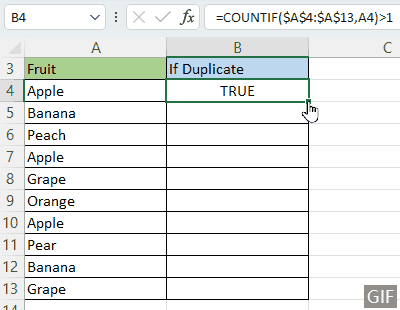##### Step 2: Count unique values or duplicate values
• For counting unique values, please use below formula
``=COUNTIF(B4:B13,"FALSE")``
• For counting duplicate values, please use below formula
``=COUNTIF(B4:B13,"TRUE")``
B4:B13 is the helper column you use formula in step 1.
Then press Enter key to get the result.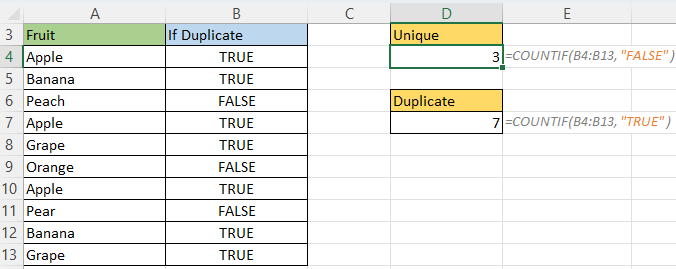If you prefer not to remember the formulas, you can utilize the Count Unique Values feature of Kutools for Excel. With just two clicks, you can obtain the count you need. Additionally, Kutools for Excel offers a wide range of formulas that allow you to efficiently perform advanced calculations with ease.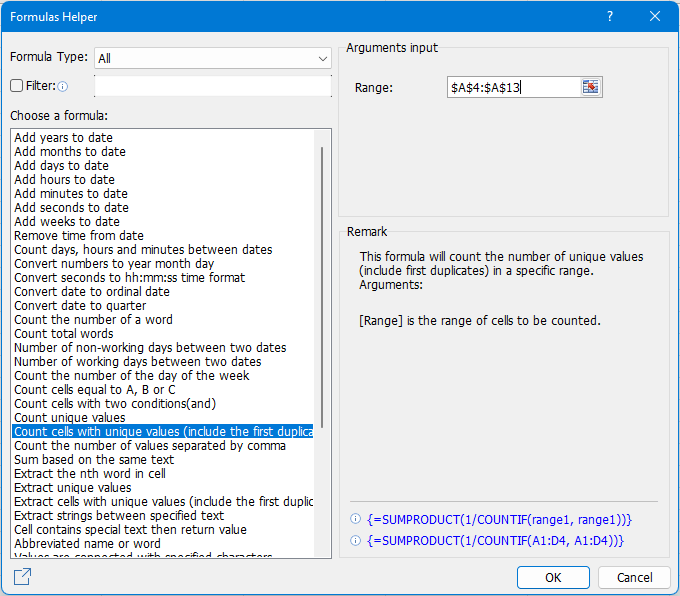• COUNTIF function is not case-sensitive.

• COUNTIF function requires an actual range, you cannot substitute an array.

• The COUNTIF function does not support non-adjacent ranges, and its syntax does not allow specifying multiple individual cells as the first parameter. If you want to count in multiple ranges, you can use a combination of several COUNTIF functions like this:
=COUNTIF(A2:A10,">0") + COUNTIF(B3,">0") + COUNTIF(C2:C5,">0").

• COUNTIF function returns wrong results when used to match strings longer than 255 characters.

• COUNTIF function returns a #VALUE error when referencing another workbook that is closed.

### Best Office Productivity Tools

Supercharge Your Spreadsheets： Experience Efficiency Like Never Before with Kutools for Excel

 Popular Features: Find/Highlight/Identify Duplicates   |  Delete Blank Rows   |  Combine Columns or Cells without Losing Data   |   Round without Formula ... Super Lookup: Multiple Criteria VLookup  |   Multiple Value VLookup  |   VLookup Across Multiple Sheets   |   Fuzzy Lookup .... Advanced Drop-down List: Quickly Create Drop Down List   |  Dependent Drop Down List   |  Multi-select Drop Down List .... Column Manager: Add a Specific Number of Columns   |   Move Columns   |   Unhide Columns   |   Compare Columns to Select Same & Different Cells ... Featured Features: Grid Focus   |  Design View   |   Big Formula Bar   |  Workbook & Sheet Manager   |  Resource Library (Auto Text)   |  Date Picker   |  Combine Worksheets   |  Encrypt/Decrypt Cells   |  Send Emails by List   |  Super Filter   |   Special Filter (filter bold/italic/strikethrough...) ... Top 15 Toolset:  12 Text Tools (Add Text, Remove Characters, ...)   |   50+ Chart Types (Gantt Chart, ...)   |   40+ Practical Formulas (Calculate age based on birthday, ...)   |   19 Insertion Tools (Insert QR Code, Insert Picture from Path, ...)   |   12 Conversion Tools (Numbers to Words, Currency Conversion, ...)   |   7 Merge & Split Tools (Advanced Combine Rows, Split Cells, ...)   |   Many More...

Kutools for Excel boasts over 300 features, ensuring that what you need is just a click away...

Supports Office/Excel 2007-2021 & newer, including 365   |   Available in 44 languages   |   Enjoy a full-featured 30-day free trial.#### Office Tab Brings Tabbed interface to Office, and Make Your Work Much Easier

• Enable tabbed editing and reading in Word, Excel, PowerPoint, Publisher, Access, Visio and Project.
• Open and create multiple documents in new tabs of the same window, rather than in new windows.
• Increases your productivity by 50%, and reduces hundreds of mouse clicks for you every day!